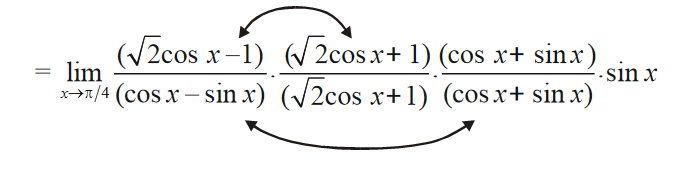# Examples On Continuity Set-1

Go back to  'LCD'

Example – 9

Find the value of f (1) if the function  \begin{align}f\left( x \right) = \frac{{{x^{m + 1}} - \left( {m + 1} \right)x + m}}{{{{\left( {x - 1} \right)}^2}}},x \ne 1\end{align}  is continuous at x =1

Solution:   We are going to encounter a lot of questions similar to the one above over the next few pages; we are given a definition of f (x) and are asked to determine f (a) so that f (x) becomes continuous at x = a. We simply evaluate $$\mathop {\lim }\limits_{x \to a} f\left( x \right)$$  and if it exists, we let f (a) equal to this limit. This ensures that the necessary and sufficient condition for continuity at x = a,  i.e

$\mathop {\lim }\limits_{x \to a} f\left( x \right) = f\left( a \right)$

is satisfied.

For this question,

\begin{align}\mathop {\lim }\limits_{x \to 1} f\left( x \right) = \mathop {\lim }\limits_{x \to 1} \frac{{{x^{m + 1}} - \left( {m + 1} \right)x + m}}{{{{\left( {x - 1} \right)}^2}}}\end{align}

\begin{align}&= \mathop {\lim }\limits_{x \to 1} \frac{{\left( {{x^{m + 1}} - x} \right) - m\left( {x - 1} \right)}}{{{{\left( {x - 1} \right)}^2}}}\\&= \mathop {\lim }\limits_{x \to 1} \frac{{x\left( {{x^m} - 1} \right) - m\left( {x - 1} \right)}}{{{{\left( {x - 1} \right)}^2}}}\\&= \mathop {\lim }\limits_{x \to 1} \frac{{x\left( {{x^{m - 1}} + {x^{m - 2}} + ... + 1} \right) - m}}{{\left( {x - 1} \right)}}\\&= \mathop {\lim }\limits_{x \to 1} \frac{{\left( {{x^m} - 1} \right) + \left( {{x^{m - 1}} - 1} \right) + ...\left( {x - 1} \right)}}{{\left( {x - 1} \right)}}\\&= m + \left( {m - 1} \right) + ... + 1\\&= \frac{{m\left( {m + 1} \right)}}{2}\end{align}

Therefore

$f\left( 1 \right) = \frac{{m\left( {m + 1} \right)}}{2}$

Example – 10

Find the value of  $$f\left( {\frac{\pi }{4}} \right)$$   if the function

$f\left( x \right) = \frac{{\sqrt 2 \cos x - 1}}{{\cot x - 1}},\,\,\,\,\,\,\,\,\,\,x \ne \frac{\pi }{4}$

is continuous at $$x = \frac{\pi }{4}$$

Solution:    This question is very similar to the previous one:

\begin{align}\mathop {\lim }\limits_{x \to {\rm{ }}\pi {\rm{ }}/{\rm{4}}\;} {\mkern 1mu} f\left( x \right) &= \mathop {\lim }\limits_{x \to {\rm{ }}\pi {\rm{ }}/{\rm{4}}\;} {\mkern 1mu} \frac{{\sqrt 2 \cos x - 1}}{{\cot x - 1}}\\&= \mathop {\lim }\limits_{x \to \pi /4\;} {\mkern 1mu} \frac{{\left( {\sqrt 2 \cos x - 1} \right) \cdot \left( {\sin x} \right)}}{{\left( {\cos x - \sin x} \right)}}\end{align}\begin{align} & =\underset{x\to {\pi }/{4}\;}{\mathop{\lim }}\,\frac{2{{\cos }^{2}}x-1}{{{\cos }^{2}}x-{{\sin }^{2}}x}\cdot \frac{\left( \cos x+\sin x \right)}{\left( \sqrt{2}\cos x+1 \right)}\cdot \sin x \\ & \\ & =\underset{x\to {\pi }/{4}\;}{\mathop{\lim }}\,\cdot \frac{\cos x+\sin x}{\sqrt{2}\cos x+1}\cdot \sin x \\ & \\ & =\,\,\frac{\frac{1}{\sqrt{2}}+\frac{1}{\sqrt{2}}}{\sqrt{2}.\frac{1}{\sqrt{2}}+1}.\frac{1}{\sqrt{2}} \\ & =\,\,\frac{1}{2} \\ \end{align}

Therefore,

$$f\left( {\frac{\pi }{4}} \right) = \frac{1}{2}$$

Example – 11

Find the values of a and b if

f\left( x \right) = \left\{ {\begin{align}&{\frac{{x\left( {1 + a\cos x} \right) - b\sin x}}{{{x^3}}},}{x \ne 0}\\&{1,\qquad\qquad\,\,\,\,\,\;\;\;\;\;\;\;\;\;} {x = 0}\end{align}} \right\}

is continuous at x = 0

Solution:    We obviously require

$\mathop {\lim }\limits_{x \to a} f\left( x \right) = f\left( 0 \right) = 1$

(Note that in all the three examples above, we have not found the LHL and RHL separately; we only determine the limit assuming it exists, or in other words assuming that LHL = RHL. We will soon encounter cases where LHL and RHL need to be separately determined)

We use the expansion series for sin x and cos x to get:

$\mathop {\lim }\limits_{x \to 0} f\left( x \right) = \mathop {\lim }\limits_{x \to 0} \frac{{x + ax\left( {1 - \frac{{{x^2}}}{{2!}} + \frac{{{x^4}}}{{4!}} - ...} \right) - b\left( {x - \frac{{{x^3}}}{{3!}} + \frac{{{x^5}}}{{5!}} - ...} \right)}}{{{x^3}}}$Now notice that (1 + ab) must be necessarily 0 otherwise \begin{align}\mathop {\lim }\limits_{x \to 0} \frac{{\left( {1 + a - b} \right)x}}{{{x^3}}}\end{align} will become infinite, Hence,

$$1\;+ a-b = \;0 \qquad \qquad \qquad \qquad \qquad \qquad ... \quad \left( i \right)$$

Also,

$$\mathop {\lim }\limits_{x \to 0} f\left( x \right) = - \left( {\frac{a}{{2!}} - \frac{b}{{3!}}} \right) = 1$$

$$6a - 2b + 12 = 0\quad \quad \quad \quad \quad \quad \quad \quad \quad \quad \quad ...\quad \left( {ii} \right)$$

Solving (i) and (ii), we get

\begin{align}a = \frac{{ - 5}}{2}\,\,\,\;\,\;\;\;\,\;\;b = \frac{{ - 3}}{2}\end{align}